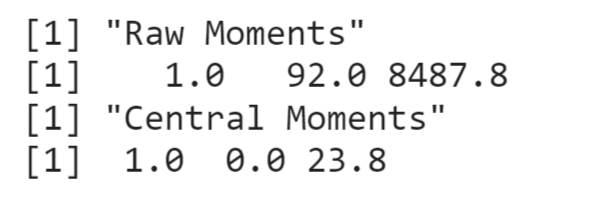Open in App
Not now

# How to Compute Raw and Central Moments Using R

• Difficulty Level : Medium
• Last Updated : 09 Oct, 2022

In this article, we are going to compute raw and central moments using R Programming Language. The moments of data used to describe the nature of the dataset are Variation, Skewness, and Kurtosis.

• Raw Moments – The moments about zero distribution are known as Raw moments.
• Central Moments – The moments about the mean of distribution are known as Central Moments.

Using R we can easily find the raw moments with the predefined package “moments”

In a moment’s package, we have all.moments() function which is used to calculate raw moments and central moments.

Syntax: all.moments(x,order.max,central=FALSE)

Where,

• x: a numeric vector of data
• order.max: the maximum order of the moments to be computed (default value is 2).
• Central: a logical value (FALSE for raw moments or TRUE for central moments)

## R

 `# install required packages ` `install.packages``(``'moments'``) ` ` `  `# load installed package ` `library``(moments) ` ` `  `# create a vector which represent marks  ` `# of student marks ` `student_marks<-``c``(98,87,96,91,85,89,93,96,99,86) ` ` `  `# Raw Moments ` `print``(``'Raw Moments'``) ` `print``(``all.moments``(student_marks)) ` ` `  `# Central Moments ` `print``(``'Central Moments'``) ` `print``(``all.moments``(student_marks,central=``TRUE``))`

Output:### Explanation:

If we observe the above R code to calculate central moments we need to make sure the third attribute of all.moments() function should be set to True because by default it’s False.

Raw Moments:-

μ′0 = 1.0

μ′1 = 92.0

μ′2 = 8487.8

Central Moments:-

μ1 = 1.0

μ2 = 0.0

μ3 = 23.8

My Personal Notes arrow_drop_up
Related Articles